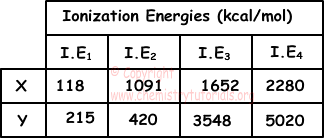EN | ES | DE | NL | RU

## Periodic Table Exam1 and Problem Solutions

Periodic Table Exam1 and  Problem Solutions

1. X, Y and Z are in same period. Using given information below, find relation between metallic properties of them.

I. Atomic number of Y is 12

II. Formula of compound produced by X and Y is YX2

III. Z-2 and X- have equal number of electrons

Solution:

I. 12Y has electron configuration: 1s22s22p63s2

Y is in 3. period and II A group. Thus, it has +2 value in compounds.

II. X in YX2 compound is in VII A group and have -1 value in compound. Atomic number of X is 17.

III. Since their number of electrons are equal, Z is in 3. period and VI A group. Relation between metallic properties of elements;

Y>Z>X

Metallic property decreases when we go from left to right in same period.

2. Which ones of the following statements are true for element having atomic number 34?

I. It is in p block

II. It is nonmetal

III. Its valence electrons are all in p orbitals

Solution:

Electron configuration of element is:

1s22s22p63s23p64s23d104p4

I. Element is in p block, since last electron is in p orbital. I is true.

II. In outer shell (4) it has 4+2=6 electrons. So it is nonmetal. II is true.

III. 4 valence electrons of element are in p orbital and 2 of them are in s orbital. So, III is false.

3. If an element has 15 filled and 1 half filled orbitals, which one of the following statements is false?

I. Atomic number of element is 31

II. It is in p block of periodic table

III. It is transition metal

IV. It is in 4. period

V. It is in III A group

Solution:

We write orbitals on electron configuration;

1s22s22p63s23p64s23d104p1

1+1+3+1+3+1+5+1= Filled orbitals and 4p1 is one half filled orbital

I. Number of electrons in neutral atom is equal to atomic number. Thus, if we sum number of electrons in given orbitals, we find atomic mass 31. I is true.

II. Since last electron of element is in p orbital, it is  p block element. II is true

III. Since its last electrons are in p orbitals, it is not transition metal. (If last electrons are in d orbitals, element becomes transition metal) III is false.

IV. Outer shell of element is 4, so it is i 4. period. IV is true.

V. It has 3 valence electrons; 2 in 4s and 1 in 4p, so it is in III A group. V is true.

4. Ionization energies of X and Y are given in the table below.Using data given in the table, find which ones of the following statements are definitely true?

I. Oxidation number of X is 1

II. Compound formed by Y and 7N is; Y3N2

III. X+ and Y+2 are isoelectronic with same noble gas

Solution:

I. Since increase in the first IE1 to second IE2 is higher than others, oxidation number of X is 1. I is true.

II. Since increase in the second IE2 to third IE3 is higher than others, oxidation number of Y is 2. In compounds Y takes +2 value. N has electron configuration;

N:1s22s22p3

N accepts 3 electrons and has value -3 in compounds. So, N and Y form following compound;

Y3N2 II is true

III. X lose 1 electron and Y 2 loses 2 electrons to have noble gas electron configuration. But, we can not definitely say they are isoelectronic.

5. Which one of the following elements has lowest first ionization energy?

I. 1s22s2

II. 1s22s22p2

III. 1s22s22p4

IV. 1s22s22p5

V. 1s22s22p63s2

Solution:

I. 1s22s2 is in 2. period and II A group

II. 1s22s22p2 is in 2. period and IV A group

III. 1s22s22p4 is in 2. period and VI A group

IV. 1s22s22p5 is in 2. period and VII A group

V. 1s22s22p63s2 is in 3. period and II A group

In a periodic table, ionization energy decreases from top to bottom and right to left. Thus, element given in V has lowest first ionization energy.

The Original Author: